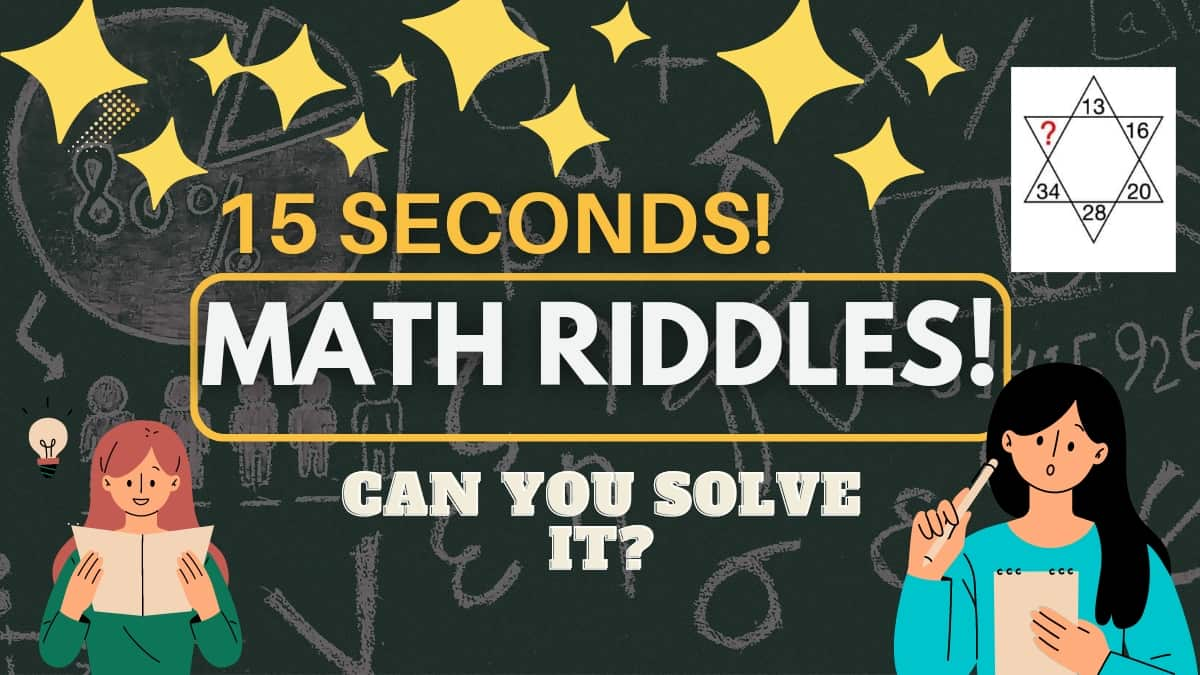# Math Riddles: Can You Find What Number Should Replace The Question, Mark? Solve This Star Puzzle In 15 Seconds!

Math Riddles are of many types, including riddles, picture puzzles, and logic puzzles but today you have a star math puzzle in front of you. Can you solve it in 15 seconds? Let's find out!Can You Find What Number Should Replace The Question, Mark? Solve This Star Puzzle In 15 Seconds!

Math Riddles: math puzzle activities are a great way to test your math skills and your IQ. It helps you have unique thinking and a creative mindset. There are a number of types of math puzzles, including riddles, picture puzzles, and logic puzzles but today you have a star math puzzle in front of you. Can you solve it in 15 seconds?

There is no comparison for the satisfaction of correctly solving an equation, now is it?

## Let's Look at the Math Puzzle Now!

Math Riddle Challenge: What Number Should Replace The Question Mark?

While solving this brain teaser puzzle, you will need to analyze the problem and need to answer it!

Is it easy?

Hint! look at the numbers placed opposite to each other

Could you figure it out?

You have 15 seconds only remember?

Time is Running out!

Tick...

Tock..

Tick..3...

2...

1...

### Time's Up!

Could you Solve it?

## Let's look at the Solution

Here you can see the star, the top number is 13 and the bottom number is 28.

## First Equation

So if we multiply 13 by 2 and add 2, the result we get is 28.

So,

## Second Equation

To check if all have the same logic, we can check for 16 and 34.

So,

### (16×2)+2=34

Hence, proved

 Did you Know? Math Puzzles can be regarded as a complete brain workout for children and adults because they should engage both the right and left sides of the brain. The right part of the brain controls creativity, emotions, and intuitive thinking, whereas the left is the analytical, systematic, and objective side.

## Third Equation

Now we can solve the final equation by using 20.

So,

## The Solution is 42

Great work!

Also Check out!

Math Riddles: Can You Solve This Atomic Math Puzzle And Find The Missing Number? Test Your IQ Here

Math Riddles: Can You Solve This Heart Puzzle In 30 Seconds?

Math Riddles: Do You Have A High IQ? Solve These Figures and Find the Missing Number!

Get the latest General Knowledge and Current Affairs from all over India and world for all competitive exams.
खेलें हर किस्म के रोमांच से भरपूर गेम्स सिर्फ़ जागरण प्ले पर